# Determining Aircraft Loaded Weight and Center of Gravity

There are various methods for determining the loaded weight and CG of an aircraft. There is the computational method as well as methods that utilize graphs and tables provided by the aircraft manufacturer.

## Computational Method

The following is an example of the computational method involving the application of basic math functions.

Aircraft Allowances:
Maximum gross weight......................3,400 pounds
CG range.............................................78–86 inches

Given:
Weight of front seat occupants.............340 pounds
Weight of rear seat occupants..............350 pounds
Fuel...........................................................75 gallons
Weight of baggage in area 1....................80 pounds

1. List the weight of the aircraft, occupants, fuel, and baggage. Remember that aviation gas (AVGAS) weighs 6 pounds per gallon and is used in this example.
2. Enter the moment for each item listed. Remember “weight x arm = moment.”
3. Find the total weight and total moment.
4. To determine the CG, divide the total moment by the total weight.

NOTE: The weight and balance records for a particular aircraft provide the empty weight and moment, as well as the information on the arm distance. [Figure 1]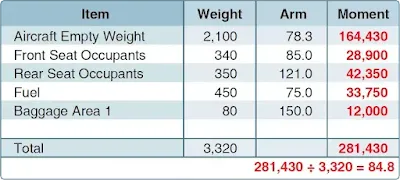Figure 1. Example of weight and balance computations

The total loaded weight of 3,320 pounds does not exceed the maximum gross weight of 3,400 pounds, and the CG of 84.8 is within the 78–86 inch range; therefore, the aircraft is loaded within limits.

## Graph Method

Another method for determining the loaded weight and CG is the use of graphs provided by the manufacturers. To simplify calculations, the moment may sometimes be divided by 100, 1,000, or 10,000. [Figures 2, 3, and 4]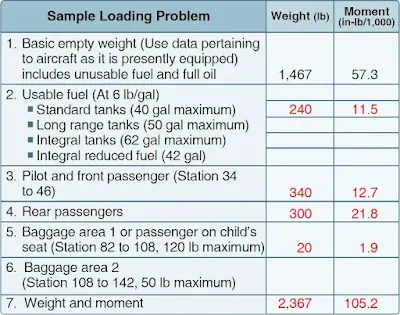Figure 2. Weight and balance data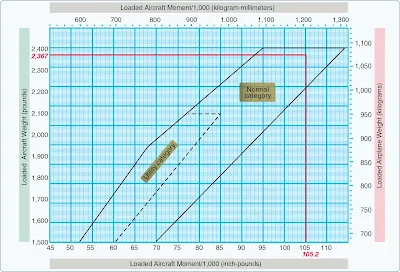Figure 4. CG moment envelope

Front seat occupants....................................340 pounds
Rear seat occupants.....................................300 pounds
Fuel ................................................................40 gallons
Baggage area 1..............................................20 pounds

The same steps should be followed in the graph method as were used in the computational method except the graphs provided will calculate the moments and allow the pilot to determine if the aircraft is loaded within limits. To determine the moment using the loading graph, find the weight and draw a line straight across until it intercepts the item for which the moment is to be calculated. Then draw a line straight down to determine the moment. (The red line on the loading graph in Figure 3 represents the moment for the pilot and front passenger. All other moments were determined the same way.) Once this has been done for each item, total the weight and moments and draw a line for both weight and moment on the CG envelope graph. If the lines intersect within the envelope, the aircraft is loaded within limits. In this sample loading problem, the aircraft is loaded within limits.

## Table Method

The table method applies the same principles as the computational and graph methods. The information and limitations are contained in tables provided by the manufacturer. Figure 5 is an example of a table and a weight and balance calculation based on that table. In this problem, the total weight of 2,799 pounds and moment of 2,278/100 are within the limits of the table.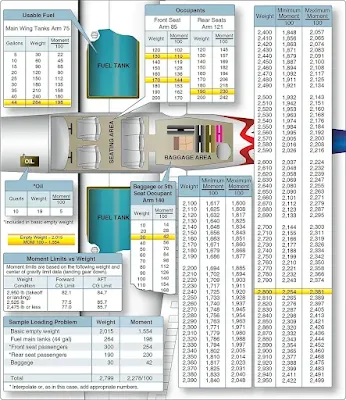Figure 5. Loading schedule placard

## Computations With a Negative Arm

Figure 6 is a sample of weight and balance computation using an aircraft with a negative arm. It is important to remember that a positive times a negative equals a negative, and a negative would be subtracted from the total moments.Figure 6. Sample weight and balance using a negative

## Computations With Zero Fuel Weight

Figure 7 is a sample of weight and balance computation using an aircraft with a zero fuel weight. In this example, the total weight of the aircraft less fuel is 4,240 pounds, which is under the zero fuel weight of 4,400 pounds. If the total weight of the aircraft without fuel had exceeded 4,400 pounds, passengers or cargo would have needed to be reduced to bring the weight at or below the max zero fuel weight.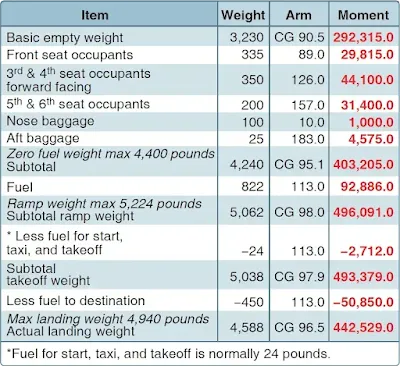Figure 7. Sample weight and balance using an aircraft with a published zero fuel weight

## Shifting, Adding, and Removing Weight

A pilot must be able to solve any problems accurately that involve the shift, addition, or removal of weight. For example, the pilot may load the aircraft within the allowable takeoff weight limit, then find that the CG limit has been exceeded. The most satisfactory solution to this problem is to shift baggage, passengers, or both. The pilot should be able to determine the minimum load shift needed to make the aircraft safe for flight. Pilots should be able to determine if shifting a load to a new location will correct an out-of-limit condition. There are some standardized calculations that can help make these determinations.

### Weight Shifting

When weight is shifted from one location to another, the total weight of the aircraft is unchanged. The total moments, however, do change in relation and proportion to the direction and distance the weight is moved. When weight is moved forward, the total moments decrease; when weight is moved aft, total moments increase. The moment change is proportional to the amount of weight moved. Since many aircraft have forward and aft baggage compartments, weight may be shifted from one to the other to change the CG. If starting with a known aircraft weight, CG, and total moments, calculate the new CG (after the weight shift) by dividing the new total moments by the total aircraft weight.

To determine the new total moments, find out how many moments are gained or lost when the weight is shifted. Assume that 100 pounds has been shifted from station 30 to station 150. This movement increases the total moments of the aircraft by 12,000 in-lb.

Moment when
at station 150 = 100 lb x 150 in = 15,000 in-lb

Moment when
at station 30 = 100 lb x 30 in = 3,000 in-lb

Moment change = [15,000 – 3,000] = 12,000 in-lb

By adding the moment change to the original moment (or subtracting if the weight has been moved forward instead of aft), the new total moments are obtained. Then determine the new CG by dividing the new moments by the total weight:

Total moments = 616,000 in-lb + 12,000 in-lb = 628,000 in-lb
CG = 628,000 in-lb = 78.5 in
8,000 lb

The shift has caused the CG to shift to station 78.5.

A simpler solution may be obtained by using a computer or calculator and a proportional formula. This can be done because the CG will shift a distance that is proportional to the distance the weight is shifted.